Page No. 1027

Math games / Math Worksheets / Division Worksheets

Download free printable division worksheets in PDF. Our math division worksheets are generated automatically, so you get an unlimited number of unique division problems with answers.

Print out division problems in PDF and use them in the classroom or for home practice.

These free division worksheets contain also a link to the online division game, so kids can practice division without printing the division worksheet.

Find our online division games here →

## Printable Division Worksheets in PDF

### Division sums

Single digit division worksheets with answers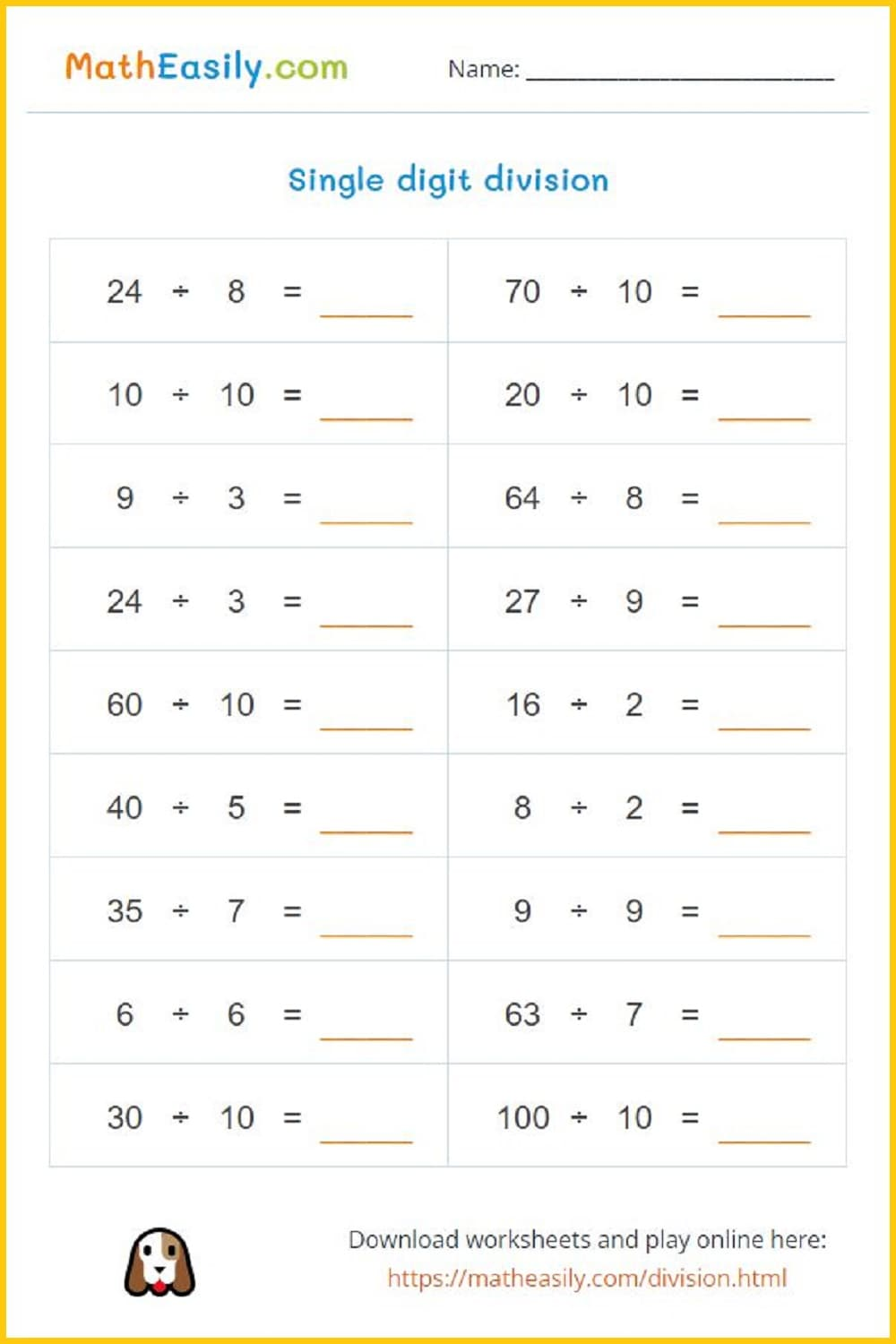Generate a new PDF

#### Division puzzles

Generate division puzzles, more than 70 pictures available.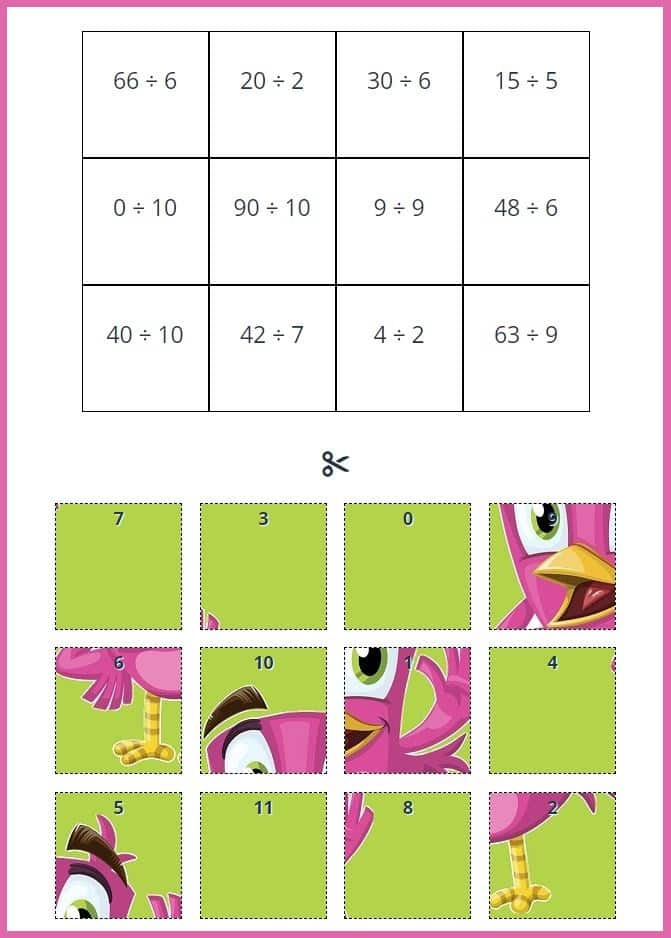Generate a new PDF

#### Picture division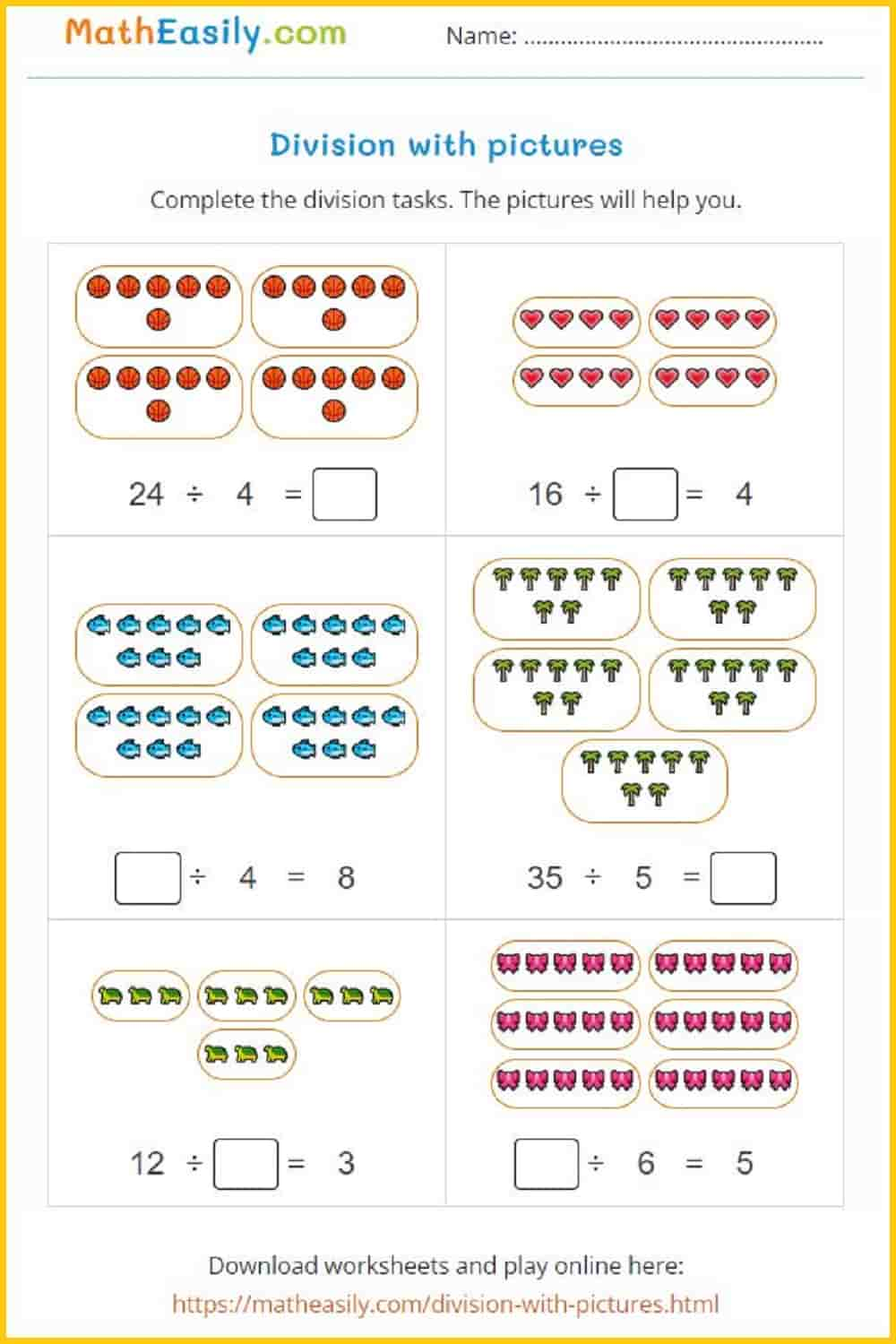Generate a new PDF

## Math division sums with answers

Our division worksheets allowing unlimited division practice opportunities. The division sums with answers can be self-checked by the students or used by teachers for grading. The advantages of automatically generated division problems include convenience, efficiency, and the ability to generate an unlimited number of unique division problems with answers.

### Multiplication and division board game

Printable division board game in PDF.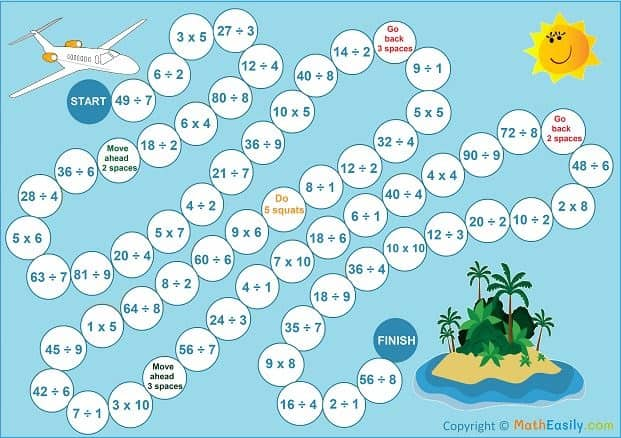### Division flash cards

Division flash cards to practice division problems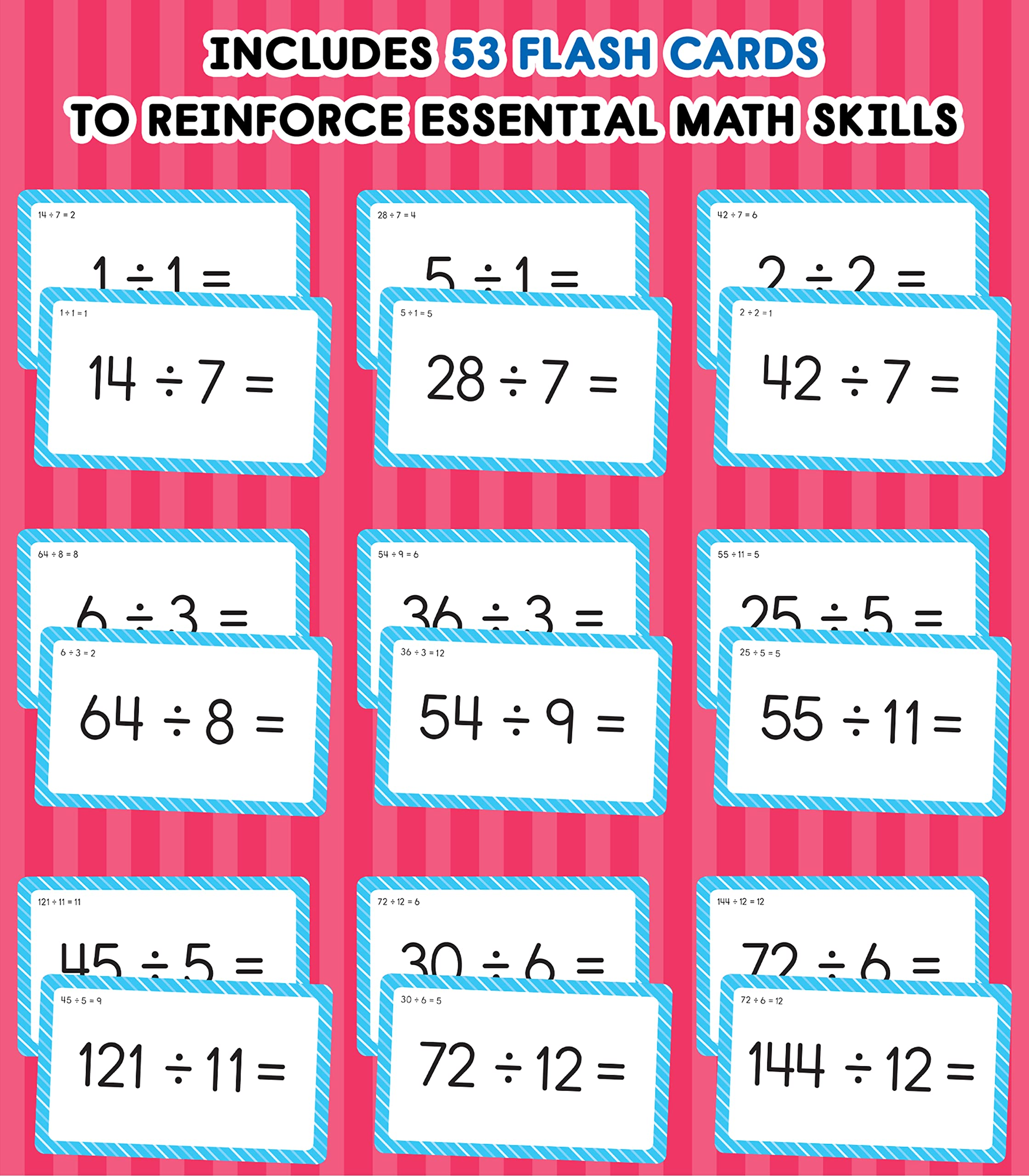### Multiplication crosswords

Generate multiplication and division crossword puzzles.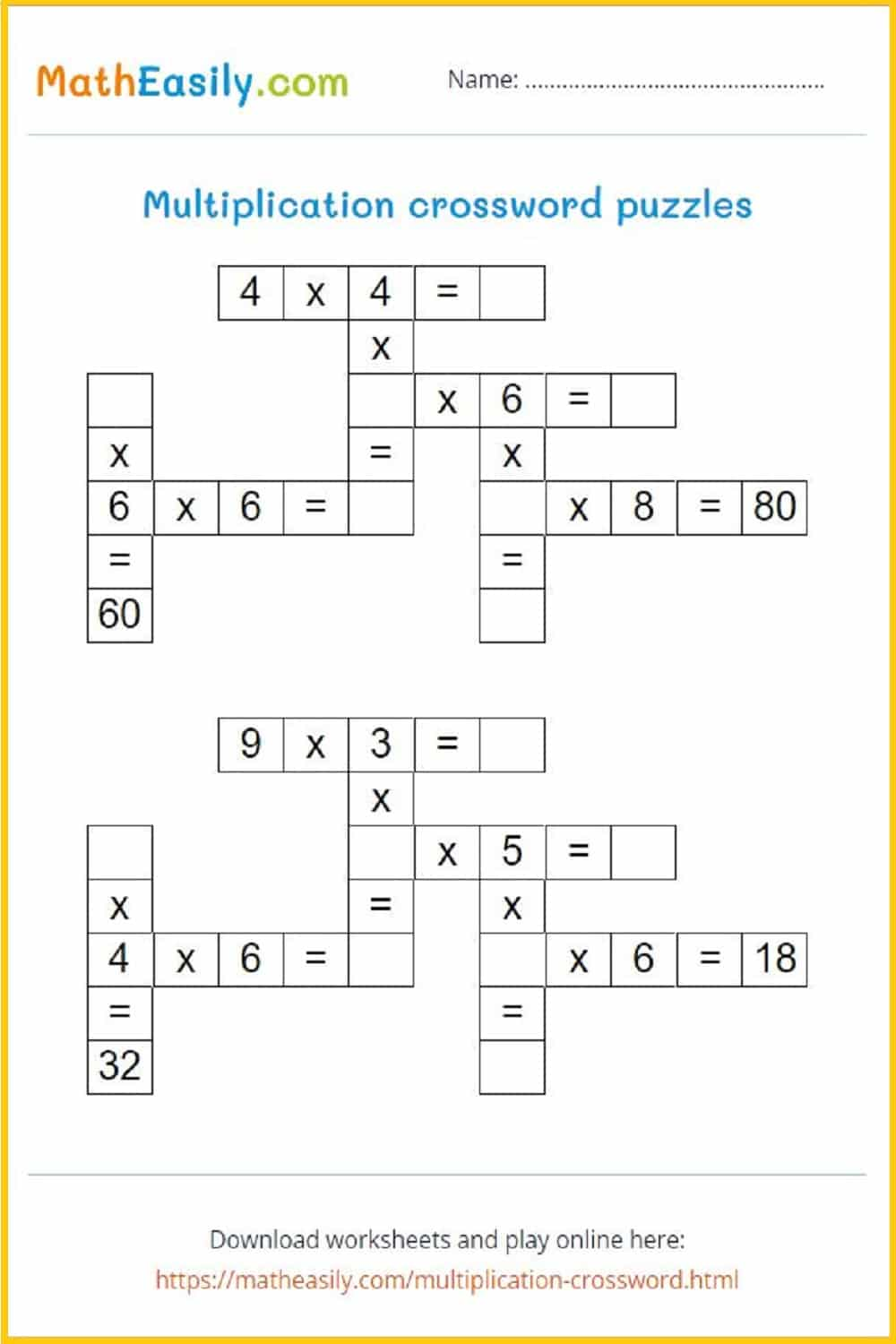Generate a new PDF

#### Division grouping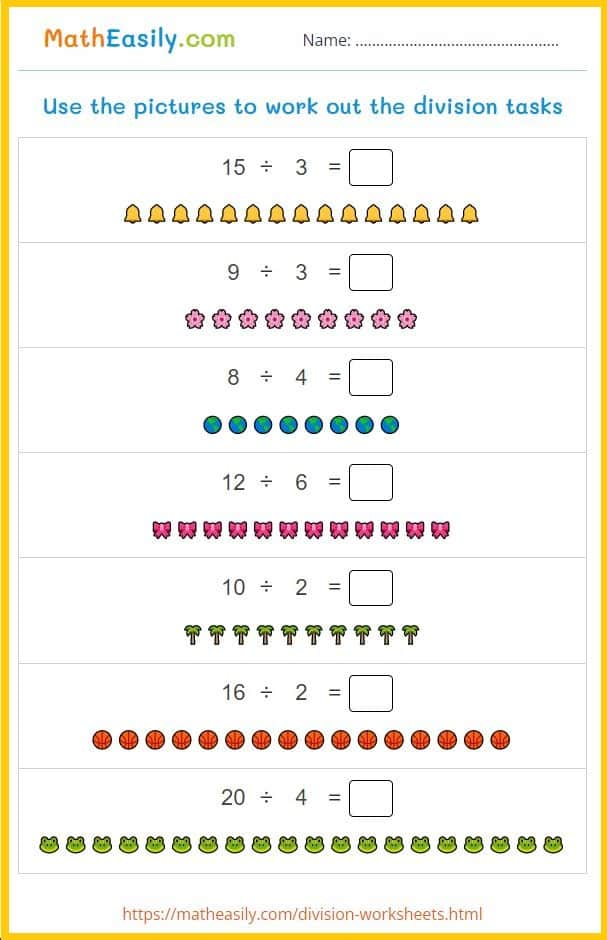Generate a new PDF

#### Factor BINGO game

Missing factor game: numbers 1 to 6.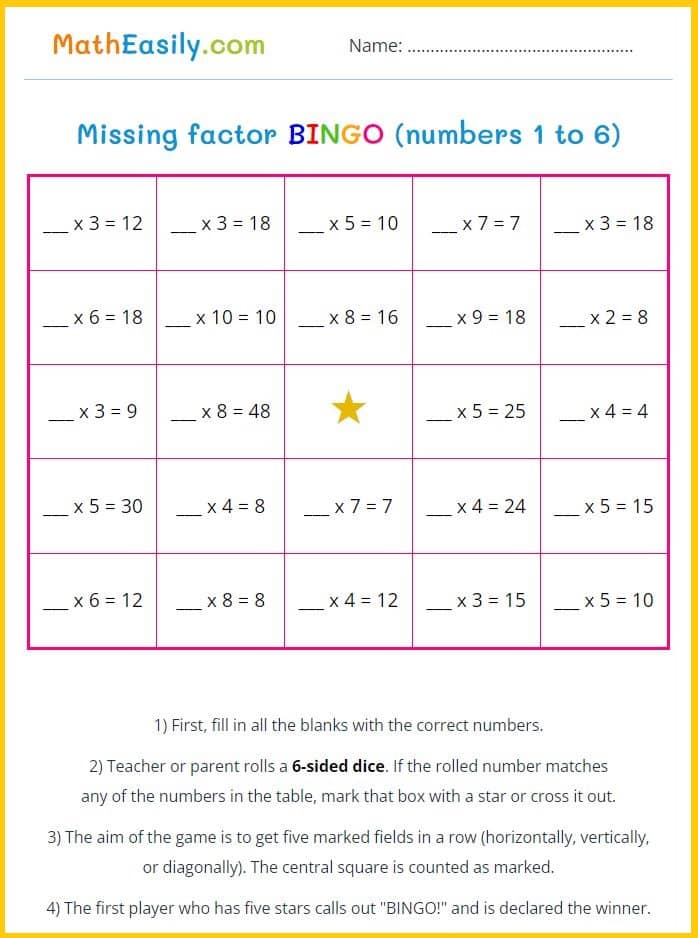Generate a new PDF

#### Missing factor game

Missing factor BINGO: numbers 1 to 10.Generate a new PDF

### Emoji maths puzzles

Puzzles with multiplication and division problemsGenerate a new PDF

### Single digit multiplication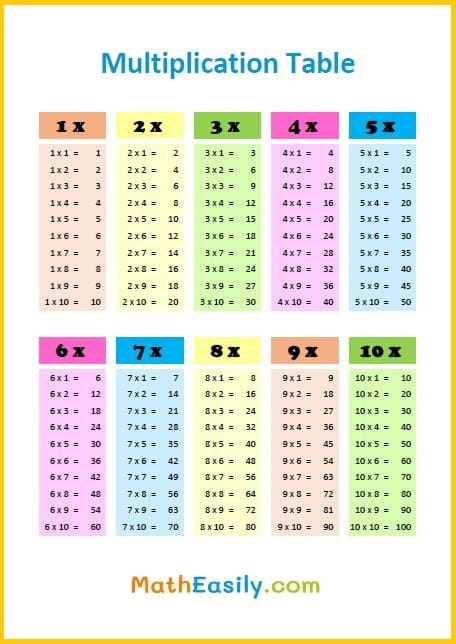##### Multiplication sheets in PDF

50 multiplication puzzles

Fun printable puzzles to practice multiplication. 50 different pictures, many multiplication tasks.

US\$ 3.00

## Practice division problems PDF

Our math division worksheets are educational resources that provide practice exercises for students to learn and master the mathematical operation of division.

These division sums include sets of division problems along with spaces for students to write in their answers.

The division worksheets are designed to increase the student's understanding and ability to perform division operations accurately and efficiently.

You may use any of these free division worksheets PDF in the classroom or for homework. Click on the math division worksheet image and download the printable PDF.

##### 100 Matchstick Puzzles in PDF

Printable math matchstick puzzles with answers. Move 1 stick to fix the equation.

US\$ 7.00

LOOK INSIDE